+
Common Core: S.ID.6b

# Residuals

Author: Sophia Tutorial
##### Description:

Calculate the residual of a given situation.

(more)

Sophia’s self-paced online courses are a great way to save time and money as you earn credits eligible for transfer to many different colleges and universities.*

No credit card required

37 Sophia partners guarantee credit transfer.

299 Institutions have accepted or given pre-approval for credit transfer.

* The American Council on Education's College Credit Recommendation Service (ACE Credit®) has evaluated and recommended college credit for 32 of Sophia’s online courses. Many different colleges and universities consider ACE CREDIT recommendations in determining the applicability to their course and degree programs.

Tutorial
what's covered
This tutorial will cover the topic of residuals, which occur when you fit a line to data points. Our discussion breaks down as follows:

1. Residuals

## 1. Residuals

When you create a best-fit line, typically it doesn't pass through all the points. The only way it would pass through all the points is if the correlation was exactly 1, which means that all the points lie exactly on a line.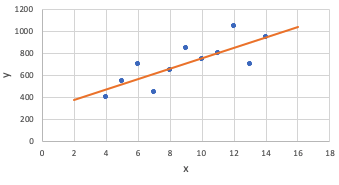Most of the time, they don't lie exactly on a line. In that case, most of the points are going to have some difference between what the line predicts and the value that they actually are. Because the line shows predictions, they'll be off a little bit from the actual values, even if only by a little.

A residual is the amount by which the predictions are off from the actual amount.

EXAMPLE

The scatterplot below shows the 1992 payrolls for the National Football League for their quarterback, who's usually their most expensive player, and for the entire team.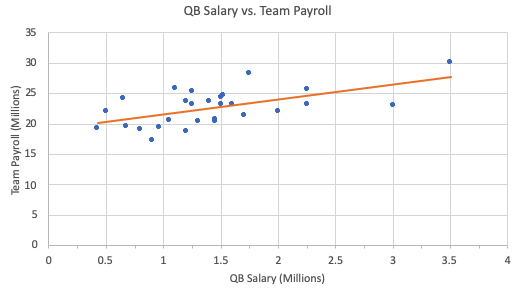The best-fit line shows the predicted payrolls of a team if the quarterback makes a certain amount of money. The predicted payroll (payroll-hat) is equal to \$18.8 million plus 3 times the quarterback salary (QB). The equation of this line is: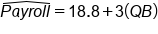Let's consider the Dallas Cowboys, circled in the scatterplot.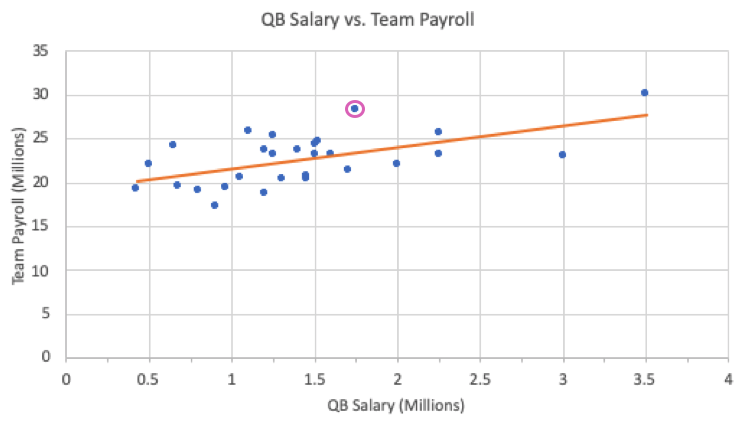They pay their quarterback \$1.75 million, and they pay the overall team \$28.394 million, which is well above what the line would predict for a team that pays their quarterback that amount of money. To find the predictive value, we can use the best-fit line equation, plug in the value of the quarterback salary for the Cowboys, and solve for the team payroll.We would predict that if a team pays their quarterback \$1.75 million, their team payroll would be \$24.05 million. We can also look at this visually to confirm this predictive value.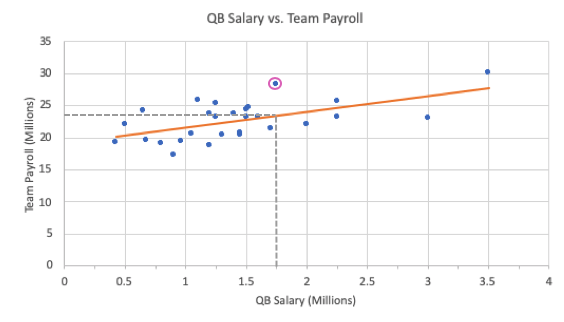However, when you look at the Dallas Cowboys' data, the actual payroll is \$28.394 million. That's over \$4 million more than the line would have predicted their payroll to be. This vertical distance between the \$28.394 million that is actually being paid versus the \$24.05 million that's being predicted is called the residual between those two values.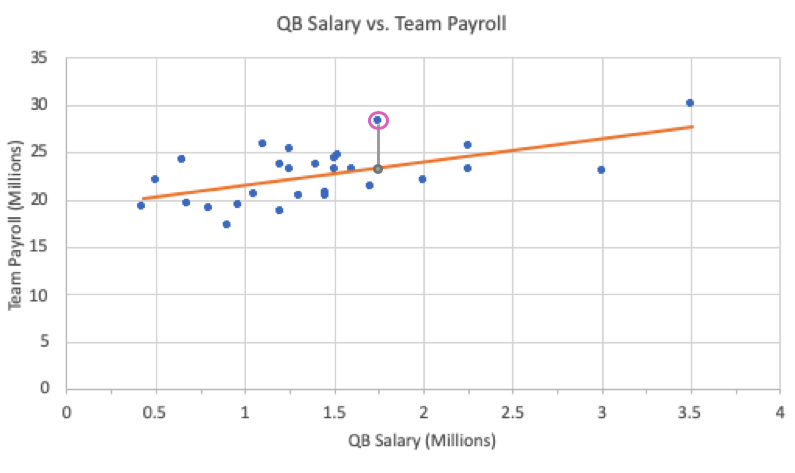The residual is calculated by taking the actual response value, y, minus the predicted response value, y-hat.

formula
Residual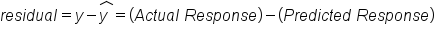So in this case, the residual for the Dallas Cowboys is calculated by taking the actual team payroll minus the predicted team payroll:In this particular problem, the residual for the Dallas Cowboys ends up being \$4.344 million. This is a positive number.

Every point has a residual value:

• If the actual response falls above the best-fit line, meaning the actual response is higher than the predicted response, the residual value is positive.
• If the actual response falls below the best-fit line, meaning the actual response is lower than the predicted response, the residual value is negative.
• If by some chance the point falls on the line, the residual value is zero.
term to know
Residual
The difference between the actual value of the response variable for a particular data point and its predicted value from the regression line.

## 2. Residual Plots

Since every point has a residual value, you can actually plot the explanatory variable vs. the residual value, as opposed to the explanatory variable vs. the response variable.

 ScatterplotExplanatoryvs.ResponseResidual PlotExplanatoryvs.Residual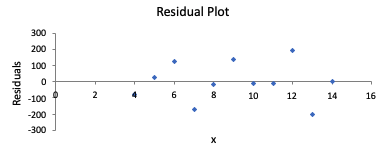The second graph, where you see how far off the predictions are, is called a residual plot. A residual plot is quite useful because it can help you evaluate whether or not a line is actually a useful predictor for the data.

A good linear model will have:

• Points above and below the line in random scatters
• No curved pattern in the residuals
• Equal variability throughout the entire residual plot.

Good Example of Linear Model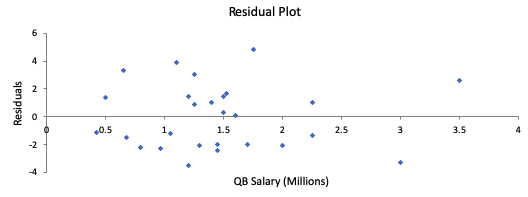This is a good choice for a best-fit line.

The points above and below the line are in random scatters, there is no curved pattern in the residual plot, and there is equal variability throughout the entire residual plot.
Bad Example: Does Not Have Random Scatter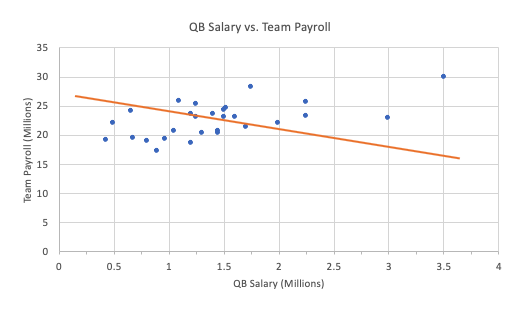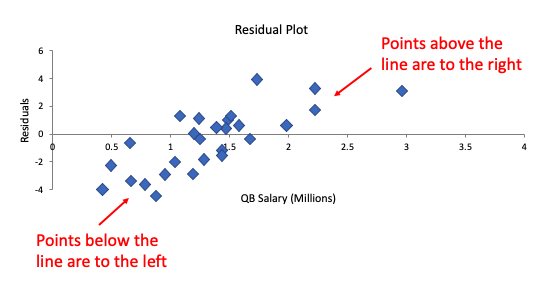This is a bad choice for a best-fit line.

Although it has points above and below as residuals, it is not randomly scattered like the original one was. There is a clear pattern that is shown on the residual. This one has points that are below only on the left, and points that are above only on the right. That's what makes this line a poor choice for a line of best fit.
Bad Example: Has a Curved Pattern in Residual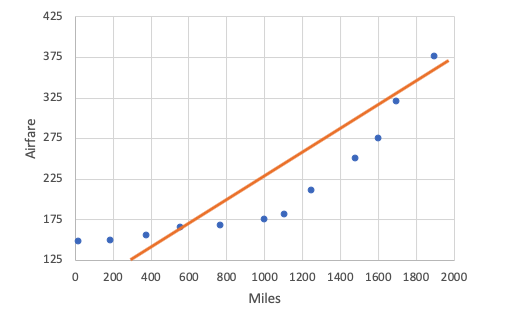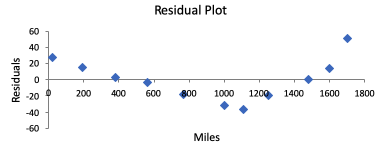This is a bad choice for a best-fit line.

Actually, a line doesn't make sense to predict this at all. You can verify that from the residual plot. What you see is a curved pattern in the residual plot. Also, it means that the scatter is not very random. What a curved pattern in the residual plot implies is that there is a better fit than a line for your data.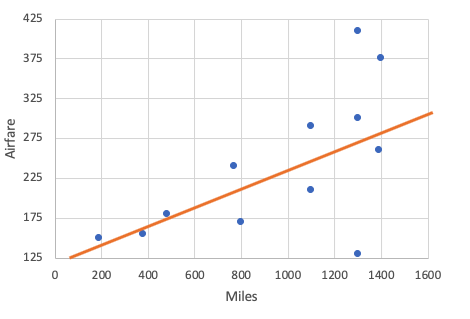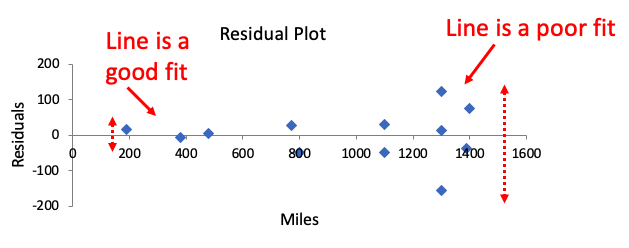This is a bad choice for a best-fit line.

This residual plot shows sort of a trumpet pattern where the variability gets wider. The line is a good fit at the beginning because the residuals are small, but it's a poor fit at the end, where the residuals are getting larger. You can also see this in the scatter plot. They're close to the line; some are fitting the line well, and others are not fitting the line.

term to know

Residual Plot
A scatter plot that plots Residuals vs. explanatory variable, as opposed to response variable vs. explanatory variable. It can be used to assess the fit of a line.

summary
Residuals are how much the data points are different than the line of best fit. They're positive if a point lies above the line, negative if it falls below the line, and zero if it falls on the line. You can use the resulting residual plot to determine if a line is actually an effective model for predicting the data.

Good luck!

Source: Adapted from Sophia tutorial by Jonathan Osters.

Terms to Know
Residual

The difference between the actual value of the response variable for a particular data point and its predicted value from the regression line.

Residual Plot

A scatter plot that plots Residuals vs. explanatory variable, as opposed to response variable vs. explanatory variable. It can be used to assess the fit of a line.

Formulas to Know
ResidualRating